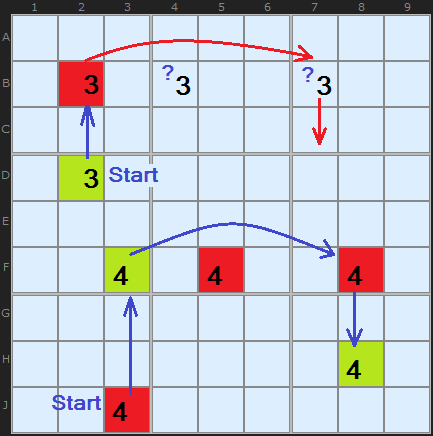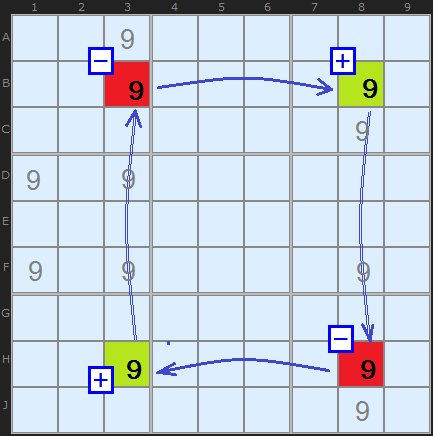Main Page - Back

From sudokuwiki.org, the puzzle solver's siteHere I clarify certain terms scattered about the strategies and put them in one place for easy reference and well as grow the theory on how chains are formed. These terms include Weak and Strong Links, or more accurately, links with weak or strong "inference". They were introduced in the X Cycles strategy but have wide application.

In this diagram I am showing two short chains. In fact, only the top one using 3's is a chain since all the component candidates are conjugate pairs. The problem with the bottom chain is that there are three 4s in row F. The red line indicates the potential link that fails because the 4s on the row are not bi-location 4s.Figure 2: Two Chains on 4 But, there is another way of thinking about chains and it directly relates to the ON/OFF states that all the candidates must have.

In the diagram I show the same two chains. The start cell is essentially the same. In the upper 3s chain the start cell D2 is ON which causes the 3 in B2 to go off. Now, since there are two remaining 3s in row B the consequence of the OFF state in B2 ends. We don't know which of B4 or B7 to turn on.

But if we change the start state to OFF, as in J3 this causes F3 to be ON. When a candidate is turned on it turns OFF ALL the other candidates in all units it can see - including the 4 in F8. So there is a consequence and the chain can continue to H8.Figure 3: Nice Loop on 9 A fuller example: Figure 3 marks out a classic X-Wing. X-Wings always contain four numbers arranged in a rectangle such that two opposite sides contain just two remaining candidates of a certain number, in this case 9. Such pairs are minimal 1-link chains, or simply bi-location pairs. If our X-Wing was a 4-link loop containing just conjugate pairs then it wouldn't be interesting - there would be no eliminations.

In Figure 3 the two pairs are at [B3,B8] and [H3,H8]. From a pair it is possible to draw two separate inferences, called weak and strong:
1. If one cell is the solution then the other cannot be - link of weak inference
2. If one cell is NOT the solution the other must be - link of strong inference
In other words, a strong link is where:

!A => B (if not A, then B) OFF implies ON

A => !B (if A, then not B) ON implies OFF

Back to the diagram. Consider the columns 3 and 8 which are part of the X-Wing and are marked with thin double lines. Because there are more than two 9s in the columns we can't draw a strong inference. We can only draw a weak inference, that is, if one of those 9s is the solution all the other 9s are eliminated.

## Strong can be Weak

So far the rough and ready distinction between Strong and Weak links is to do with how many candidates are in a unit — namely, Strong links are formed when only two are present, while three or more imply a Weak link. However, this is not the case.

From a strong link we can infer that
if not A, then B

From a weak link, we can infer only that
if A then not B, C, D according to how many candidates there are in a unit

However, the following is also true that for a strong link:
if A, then not B

So, some Strong links can be reversed to give us a "link with weak inference" - if the occasion calls for it. It is perfectly logical to assert on a unit with two candidates of X both:

• If Not A then B (!A =>B)
• If A then Not B (A => !B)

In Figure 4 we have an array of 6 candidates on a board. A number of strategies can show that the 6 on H9 can be eliminated. I have coloured some cells using Singles Chains Rule 4 which link up some pairs on the board - either all of the yellow cells will be 6 or all of the cyan cells will be 6. Since H9 can see C9 (yellow) and H5 (cyan) it cannot be a 6 since it can see cells with both colours.